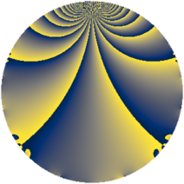# Properties

 Label 1369.2.aLevel $1369$ Weight $2$ Character orbit 1369.a Rep. character $\chi_{1369}(1,\cdot)$ Character field $\Q$ Dimension $94$ Newform subspaces $15$ Sturm bound $234$ Trace bound $6$

# Related objects

## Defining parameters

 Level: $$N$$ $$=$$ $$1369 = 37^{2}$$ Weight: $$k$$ $$=$$ $$2$$ Character orbit: $$[\chi]$$ $$=$$ 1369.a (trivial) Character field: $$\Q$$ Newform subspaces: $$15$$ Sturm bound: $$234$$ Trace bound: $$6$$ Distinguishing $$T_p$$: $$2$$, $$3$$

## Dimensions

The following table gives the dimensions of various subspaces of $$M_{2}(\Gamma_0(1369))$$.

Total New Old
Modular forms 135 129 6
Cusp forms 98 94 4
Eisenstein series 37 35 2

The following table gives the dimensions of the cuspidal new subspaces with specified eigenvalues for the Atkin-Lehner operators and the Fricke involution.

$$37$$Dim
$$+$$$$43$$
$$-$$$$51$$

## Trace form

 $$94 q + 2 q^{2} + 2 q^{3} + 78 q^{4} + 2 q^{5} - 6 q^{6} + 2 q^{7} + 56 q^{9} + O(q^{10})$$ $$94 q + 2 q^{2} + 2 q^{3} + 78 q^{4} + 2 q^{5} - 6 q^{6} + 2 q^{7} + 56 q^{9} - 4 q^{10} + 2 q^{11} + 8 q^{12} + 6 q^{13} - 2 q^{14} - 6 q^{15} + 42 q^{16} - 6 q^{17} + 12 q^{18} - 2 q^{19} + 4 q^{20} - 2 q^{21} - 10 q^{22} - 8 q^{23} + 30 q^{25} - 4 q^{26} + 14 q^{27} + 8 q^{28} + 20 q^{30} + 8 q^{31} - 8 q^{32} - 18 q^{33} + 8 q^{34} - 2 q^{35} - 10 q^{36} - 34 q^{38} - 2 q^{39} + 8 q^{40} + 20 q^{41} + 6 q^{42} - 10 q^{43} + 16 q^{44} + 12 q^{45} + 6 q^{46} + 6 q^{47} - 8 q^{48} - 2 q^{50} - 6 q^{51} - 4 q^{52} + 4 q^{53} - 18 q^{54} - 10 q^{55} - 2 q^{57} + 20 q^{58} - 20 q^{59} - 12 q^{60} - 6 q^{62} + 4 q^{63} - 14 q^{64} + 30 q^{66} + 12 q^{68} + 12 q^{70} + 10 q^{71} - 8 q^{73} - 24 q^{75} + 4 q^{76} + 2 q^{77} - 50 q^{78} + 6 q^{79} - 8 q^{80} - 58 q^{81} - 18 q^{82} + 16 q^{83} + 4 q^{85} + 6 q^{86} + 24 q^{87} - 10 q^{89} - 14 q^{90} - 6 q^{91} + 8 q^{92} - 8 q^{93} - 18 q^{94} + 4 q^{95} + 24 q^{96} - 12 q^{97} - 12 q^{98} + 46 q^{99} + O(q^{100})$$

## Decomposition of $$S_{2}^{\mathrm{new}}(\Gamma_0(1369))$$ into newform subspaces

Label Dim $A$ Field CM Traces A-L signs $q$-expansion
$a_{2}$ $a_{3}$ $a_{5}$ $a_{7}$ 37
1369.2.a.a $1$ $10.932$ $$\Q$$ None $$-2$$ $$1$$ $$-2$$ $$3$$ $-$ $$q-2q^{2}+q^{3}+2q^{4}-2q^{5}-2q^{6}+\cdots$$
1369.2.a.b $1$ $10.932$ $$\Q$$ None $$-1$$ $$0$$ $$-1$$ $$2$$ $+$ $$q-q^{2}-q^{4}-q^{5}+2q^{7}+3q^{8}-3q^{9}+\cdots$$
1369.2.a.c $1$ $10.932$ $$\Q$$ None $$0$$ $$1$$ $$0$$ $$-1$$ $+$ $$q+q^{3}-2q^{4}-q^{7}-2q^{9}+3q^{11}+\cdots$$
1369.2.a.d $1$ $10.932$ $$\Q$$ None $$1$$ $$0$$ $$1$$ $$2$$ $+$ $$q+q^{2}-q^{4}+q^{5}+2q^{7}-3q^{8}-3q^{9}+\cdots$$
1369.2.a.e $1$ $10.932$ $$\Q$$ None $$2$$ $$-3$$ $$2$$ $$-1$$ $+$ $$q+2q^{2}-3q^{3}+2q^{4}+2q^{5}-6q^{6}+\cdots$$
1369.2.a.f $1$ $10.932$ $$\Q$$ None $$2$$ $$1$$ $$2$$ $$3$$ $-$ $$q+2q^{2}+q^{3}+2q^{4}+2q^{5}+2q^{6}+\cdots$$
1369.2.a.g $2$ $10.932$ $$\Q(\sqrt{3})$$ None $$-2$$ $$2$$ $$4$$ $$0$$ $-$ $$q-q^{2}+(1-\beta )q^{3}-q^{4}+(2+\beta )q^{5}+\cdots$$
1369.2.a.h $2$ $10.932$ $$\Q(\sqrt{3})$$ None $$2$$ $$2$$ $$-4$$ $$0$$ $-$ $$q+q^{2}+(1+\beta )q^{3}-q^{4}+(-2+\beta )q^{5}+\cdots$$
1369.2.a.i $3$ $10.932$ $$\Q(\zeta_{18})^+$$ None $$-3$$ $$0$$ $$3$$ $$0$$ $+$ $$q+(-1+\beta _{1})q^{2}-\beta _{2}q^{3}+(1-2\beta _{1}+\cdots)q^{4}+\cdots$$
1369.2.a.j $3$ $10.932$ $$\Q(\zeta_{18})^+$$ None $$0$$ $$-3$$ $$0$$ $$-6$$ $+$ $$q+\beta _{1}q^{2}+(-1-\beta _{1}+\beta _{2})q^{3}+\beta _{2}q^{4}+\cdots$$
1369.2.a.k $3$ $10.932$ $$\Q(\zeta_{18})^+$$ None $$0$$ $$-3$$ $$0$$ $$-6$$ $+$ $$q-\beta _{1}q^{2}+(-1-\beta _{1}+\beta _{2})q^{3}+\beta _{2}q^{4}+\cdots$$
1369.2.a.l $3$ $10.932$ $$\Q(\zeta_{18})^+$$ None $$3$$ $$0$$ $$-3$$ $$0$$ $+$ $$q+(1-\beta _{1})q^{2}-\beta _{2}q^{3}+(1-2\beta _{1}+\beta _{2})q^{4}+\cdots$$
1369.2.a.m $18$ $10.932$ $$\mathbb{Q}[x]/(x^{18} - \cdots)$$ None $$0$$ $$6$$ $$0$$ $$12$$ $-$ $$q+(\beta _{1}-\beta _{13})q^{2}+(-\beta _{7}+\beta _{14})q^{3}+\cdots$$
1369.2.a.n $27$ $10.932$ None $$-9$$ $$-1$$ $$-17$$ $$-3$$ $+$
1369.2.a.o $27$ $10.932$ None $$9$$ $$-1$$ $$17$$ $$-3$$ $-$

## Decomposition of $$S_{2}^{\mathrm{old}}(\Gamma_0(1369))$$ into lower level spaces

$$S_{2}^{\mathrm{old}}(\Gamma_0(1369)) \cong$$ $$S_{2}^{\mathrm{new}}(\Gamma_0(37))$$$$^{\oplus 2}$$